0

Find a Corresponding Node of a Binary Tree in a Clone of That Tree

Difficulty: EASY
Avg. time to solve
20 min

Problem Statement

Note:

1. ‘cloneTree’ is an exact copy of the ‘originalTree’.
2. All nodes in ‘originalTree’ are distinct.
3. The given node in the ‘originalTree’ will not be  NULL.
4. You cannot change any of the two given trees.

Input Format:

The first line contains an integer 'T' which denotes the number of test cases or queries to be run.

The first line of each test case contains elements of the tree in the level order form. The line consists of values of nodes separated by a single space. In case a node is null, we take -1 in its place.

The second line contains an integer ‘K’ which denotes the value of the reference node in ‘originalTree’.

For example, the level order input for the tree depicted in the below image.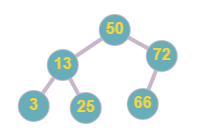50
13 72
3 25 66 -1
-1 -1 -1 -1 -1 -1

Explanation :

Level 1 :
The root node of the tree is 50

Level 2 :
Left child of 50 = 13
Right child of 50 = 72

Level 3 :
Left child of 13 = 3
Right child of 13 = 25
Left child of 72 = 66
Right child of 72 =  ‘Null’

Level 4 :
All children are ‘Null’

The first not-null node(of the previous level) is treated as the parent of the first two nodes of the current level. The second not-null node (of the previous level) is treated as the parent node for the next two nodes of the current level and so on.
The input ends when all nodes at the last level are null(-1).

Note :

The above format was just to provide clarity on how the input is formed for a given tree.
The sequence will be put together in a single line separated by a single space. Hence, for the above-depicted tree, the input will be given as:
50 13 72 3 25 66 -1 -1 -1 -1 -1 -1 -1

Output Format:

For each test case, the output will be “1” if you have returned the correct node in ‘cloneTree’, else it will be “0”.

The output of each test case will be printed in a separate line.

Note :

You do not need to input or print anything, and it has already been taken care of. Just implement the given function.

Constraints:

1 <= T <= 5
1 <= N <= 3000
1 <= data <= 10 ^ 9
1 <= K <=  10 ^ 9

Where ‘data’ is the value of the nodes of the given binary tree, and ‘K’ represents the value of the given reference node in ‘originalTree’.

For a single test case, all given ‘data’ are distinct from each other and ‘K’ is equal to one given of ‘data’.

Time Limit: 1 Sec

Sample Input 1:

2
1 -1 3 -1 4 -1 -1
4
50 -1 -1
50

1
1

Explanation Of Sample Input 1:

For the first test case, the given tree is

Original tree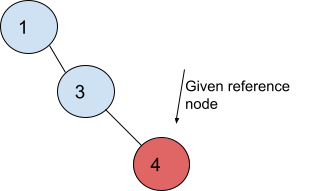Clone Tree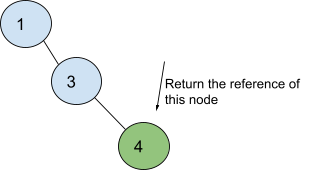The given tree in the second test case is.

Original tree.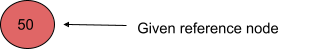Clone Tree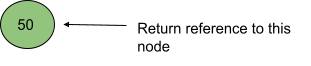Sample Input 2:

1
10 2 12 1 3 -1 13 -1 -1 -1 -1 -1 -1
2

1

Explanation Of Sample Input 2:

For the first test case, the given tree is

Original tree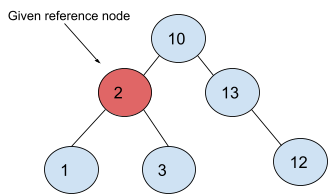Clone Tree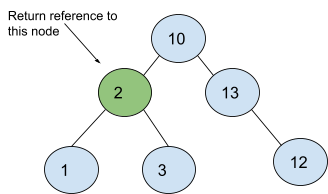Console